All-In-One Calculator is a lightweight, clean and easy to use calculator and converter pack.

Containing over 50 calculators and unit converters packed in with a scientific calculator, it’s the only math app you will ever need from now on on your device.

It’s a great calculator for school and homework, but not only. If you’re a scholar, student, teacher. builder, handyman, contractor, etc.. and you need an all in one tool for your device, give this one a try. You will never be disappointed.

Features
• Over 50 Calculators and Unit converters
• Scientific calculator with history
• Currency converter with 160 currencies, available offline
• Supports math formulas as input
• Instant results
• Integrated search
• Favorite list for quick access
• Tablet support

Languages: English (currently looking for translators)

Complete list of all the calculators and unit converters
Algebra
• Percentage calculator
• Proportion calculator
• Average calculator – arithmetic, geometric and harmonic means
• Equation solver – linear, quadratic and equation system.
• Combinations and permutations
• Decimal to fraction
• Fraction simplifier
• Greatest common factor & Lowest common multiple calculator
• Random number generator

Geometry
• Area / perimeter calculator for square, rectangle, parallelogram, trapezoid, rhombus, triangle, pentagon, hexagon, circle, circle arc, ellipse
• Volume calculator for cube, rect. prism, square pyramid, sq. pyramid frustum, trapezoidal footing, cylinder, cone, conical frustum, sphere, spherical cap, spherical frustum, ellipsoid.
• Right triangle calculator
• Heron’s formula (solve a triangle knowing the side lengths)
• Circle solver

Unit converters
• Acceleration converter
• Angle converter
• Length converter
• Energy converter
• Force converter
• Torque converter
• Area converter
• Volume converter
• Volumetric flow converter
• Weight converter
• Temperature converter
• Pressure converter
• Power converter
• Speed converter
• Mileage converter
• Time converter
• Digital storage converter
• Data transfer speed converter
• Numeric base converter
• Roman numerals converter
• Shoe size converter

Finance
• Currency converter with 161 currencies available offline
• VAT calculator
• Tip calculator
• Loan calculator
• Electricity cost calculator
• Cost of smoking calculator

Health
• Body mass index – BMI
• Daily calories burn
• Body fat percentage

Engineering
• Ohm’s law calculator – voltage, current, resistance and power
• Speed/Distance/Time
• Cylinder force calculator
• Resistance calculator
• Density calculator

Miscellaneous
• Elapsed time calculator

WHAT’S NEW

Version 1.1.5
Various minor issues fixed
Added something that was not here before, but in a very nice and decent wayMod Info:

• Pro / Paid features unlocked;
• Disabled / Removed unwanted Permissions + Receivers + Providers + Services;
• Optimized and zipaligned graphics and cleaned resources for fast load;
• Ads Permissions / Services / Providers removed from Android.manifest;
• Google Play Store install package check disabled;
• Debug code removed;
• Remove default .source tags name of the corresponding java files;
• Analytics / Crashlytics / Firebase disabled;
• AOSP compatible mode;
• Languages: Full Multi Languages;
• CPUs: universal architecture;
• Screen DPIs: 160dpi, 240dpi, 320dpi, 480dpi, 640dpi;
• Original package signature changed.

Screenshots

•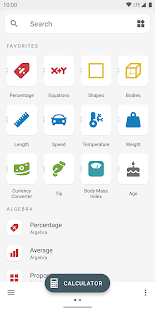•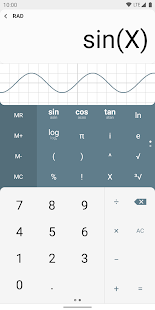•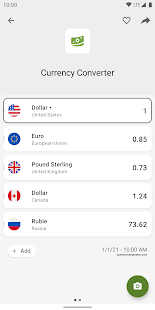•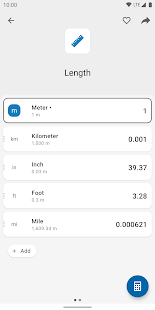•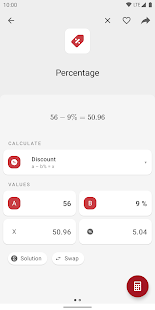•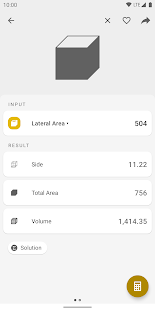•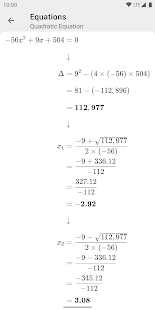•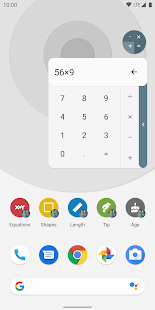•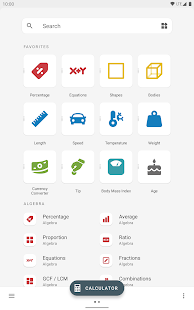•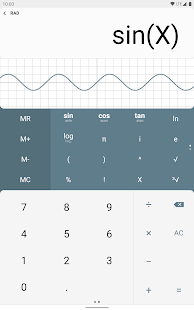••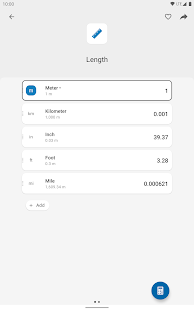•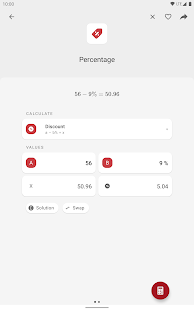•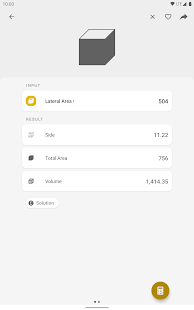•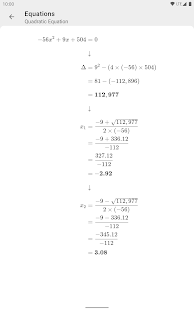•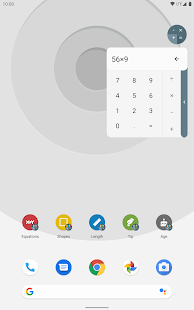•••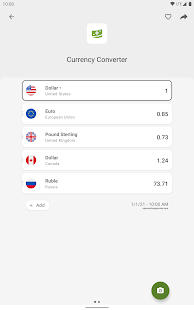•••••